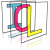Image Component Library (ICL)
icl::core::Line32f Class Reference

The ICLs abstract line class describing a line from Point "start" to Point "end". More...

`#include <Line32f.h>`

## Public Member Functions

Line32f (utils::Point32f start=utils::Point::null, utils::Point32f end=utils::Point::null)
Creates a new line from point "start" to point "end". More...

Line32f (utils::Point32f start, float angle, float length)
Creates a new line by given polar coordinates. More...

Line32f (const Line &l)
Creates a line by a given integer line. More...

Line32f operator+ (const utils::Point32f &p) const
translates a line by a given vector More...

Line32f operator- (const utils::Point32f &p) const
translates a line by a given vector (negative direction) More...

float length () const
calculates the euclidean norm of this line More...

float getAngle () const
returns line angle [ atan2(dy,dx) ] More...

utils::Point32f getCenter () const
returns the lines center point [ (start+end)/2 ] More...

bool intersects (const core::Line32f &other, utils::Point32f *p=0, float *dstr=0, float *dsts=0) const
return whether the line intersects with the given other line More...

std::vector< utils::Pointsample (const utils::Rect &limits=utils::Rect::null) const
samples this line from start to end point regarding the given limiting rect More...

void sample (std::vector< int > &xs, std::vector< int > &ys, const utils::Rect &limits=utils::Rect::null) const
samples this line from start to end point regarding the given limiting rect More...

void swap ()
swaps the lines start and end point internally More...

## Public Attributes

utils::Point32f start
start point of this line More...

utils::Point32f end
end point of this line More...

## Static Public Attributes

static const Line32f null
Null line of length 0 with and and end point 0. More...

## Detailed Description

The ICLs abstract line class describing a line from Point "start" to Point "end".

icl::Line

## ◆ Line32f() [1/3]

 icl::core::Line32f::Line32f ( utils::Point32f start = `utils::Point::null`, utils::Point32f end = `utils::Point::null` )
inline

Creates a new line from point "start" to point "end".

Parameters
 start start point end end point

## ◆ Line32f() [2/3]

 icl::core::Line32f::Line32f ( utils::Point32f start, float angle, float length )

Creates a new line by given polar coordinates.

Parameters
 start start point of the line angle angle of the line length length of the line

## ◆ Line32f() [3/3]

 icl::core::Line32f::Line32f ( const Line & l )

Creates a line by a given integer line.

Parameters
 l interger line

## ◆ getAngle()

 float icl::core::Line32f::getAngle ( ) const

returns line angle [ atan2(dy,dx) ]

## ◆ getCenter()

 utils::Point32f icl::core::Line32f::getCenter ( ) const

returns the lines center point [ (start+end)/2 ]

## ◆ intersects()

 bool icl::core::Line32f::intersects ( const core::Line32f & other, utils::Point32f * p = `0`, float * dstr = `0`, float * dsts = `0` ) const

return whether the line intersects with the given other line

Optionally, the actual intersection point can be calculated and stored into a non-null p argument. dstr and dsts are filled with the interpolation factors between start and end for the actual intersection point if not null. If there is no intersection, p, dstr and dsts are not used

## ◆ length()

 float icl::core::Line32f::length ( ) const

calculates the euclidean norm of this line

Returns
length of the line

## ◆ operator+()

 Line32f icl::core::Line32f::operator+ ( const utils::Point32f & p ) const
inline

translates a line by a given vector

Parameters
 p translation vector
Returns
the translated line

## ◆ operator-()

 Line32f icl::core::Line32f::operator- ( const utils::Point32f & p ) const
inline

translates a line by a given vector (negative direction)

Parameters
 p translation vector
Returns
the translated line

## ◆ sample() [1/2]

 std::vector icl::core::Line32f::sample ( const utils::Rect & limits = `utils::Rect::null` ) const

samples this line from start to end point regarding the given limiting rect

Parameters
 limits each line point is check for being inside of this rect the eases working e.g. on image planes, that have an finite extend. If the limits rect has width*height == 0, the limits are not regarded.
Returns
vector of line Points

## ◆ sample() [2/2]

 void icl::core::Line32f::sample ( std::vector< int > & xs, std::vector< int > & ys, const utils::Rect & limits = `utils::Rect::null` ) const

samples this line from start to end point regarding the given limiting rect

This function works essentially like the above function. In this case, the result is not returned, but it is stored into the given vector references.

Parameters
 xs destination vector for x-coordinates (filled using push_back, so it is not cleared before it is filled) ys as xs but for the y-coordinates limits (see above)

## ◆ swap()

 void icl::core::Line32f::swap ( )
inline

swaps the lines start and end point internally

## ◆ end

 utils::Point32f icl::core::Line32f::end

end point of this line

## ◆ null

 const Line32f icl::core::Line32f::null
static

Null line of length 0 with and and end point 0.

## ◆ start

 utils::Point32f icl::core::Line32f::start

start point of this line

The documentation for this class was generated from the following file:
• /Users/alneuman/vm/icl/ICLCore/src/ICLCore/Line32f.h